# ML Aggarwal Class 10 Solutions for ICSE Maths Chapter 13 Similarity Ex 13.1

## ML Aggarwal Class 10 Solutions for ICSE Maths Chapter 13 Similarity Ex 13.1

These Solutions are part of ML Aggarwal Class 10 Solutions for ICSE Maths. Here we have given ML Aggarwal Class 10 Solutions for ICSE Maths Chapter 13 Similarity Ex 13.1

More Exercises

Question 1.
State which pairs of triangles in the figure given below are similar. Write the similarity rule used and also write the pairs of similar triangles in symbolic form (all lengths of sides are in cm):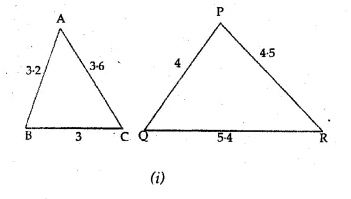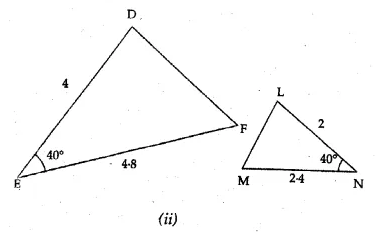Solution:
Given
(i) In ∆ABC and PQR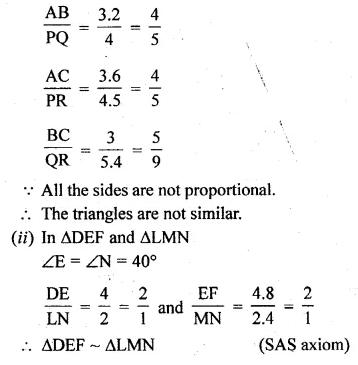Question 2.
It is given that ∆DEF ~ ∆RPQ. Is it true to say that ∠D = ∠R and ∠F = ∠P ? Why?
Solution:
∆DEF ~ ∆RPQ
∠D = ∠R and ∠F = ∠Q not ∠P
No, ∠F ≠ ∠P

Question 3.
If in two right triangles, one of the acute angle of one triangle is equal to an acute angle of the other triangle, can you say that the two triangles are similar? Why?
Solution:
In two right triangles,
one of the acute angles of the one triangle is
equal to an acute angle of the other triangle.
The triangles are similar. (AAA axiom)

Question 4.
In the given figure, BD and CE intersect each other at the point P. Is ∆PBC ~ ∆PDE? Give reasons for your answer.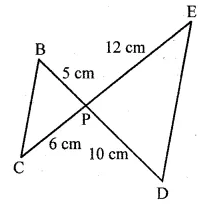Solution:
In the given figure, two line segments intersect each other at P.
In ∆BCP and ∆DEP
∠BPC = ∠DPE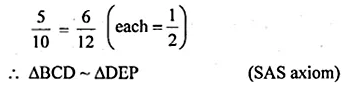Question 5.
It is given that ∆ABC ~ ∆EDF such that AB = 5 cm, AC = 7 cm, DF = 15 cm and DE = 12 cm.
Find the lengths of the remaining sides of the triangles.
Solution:
∆ABC ~ ∆EDF
AB = 5 cm, AC = 7 cm, DF = 15 cm and DE = 12 cm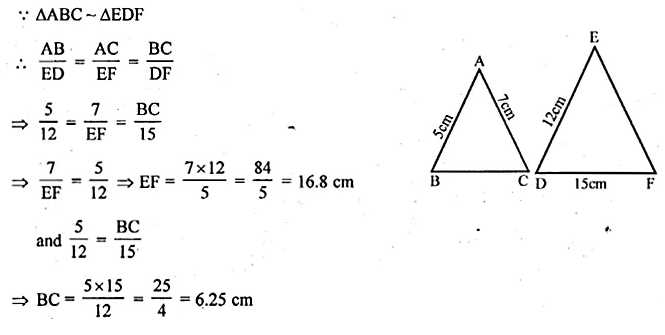Question 6.
(a) If ∆ABC ~ ∆DEF, AB = 4 cm, DE = 6 cm, EF = 9 cm and FD = 12 cm, then find the perimeter of ∆ABC.
(b) If ∆ABC ~ ∆PQR, Perimeter of ∆ABC = 32 cm, perimeter of ∆PQR = 48 cm and PR = 6 cm, then find the length of AC.
Solution:
(a) ∆ABC ~ ∆DEF
AB = 4 cm, DE = 6 cm, EF = 9 cm and FD = 12 cm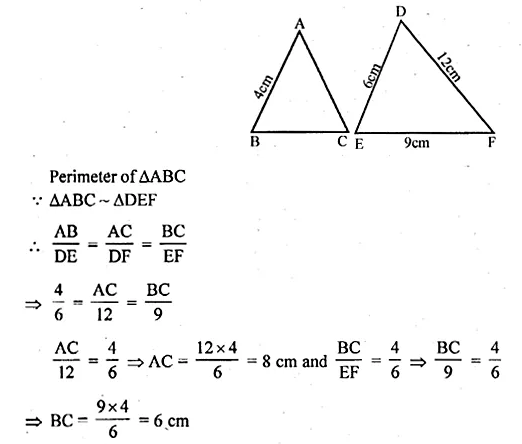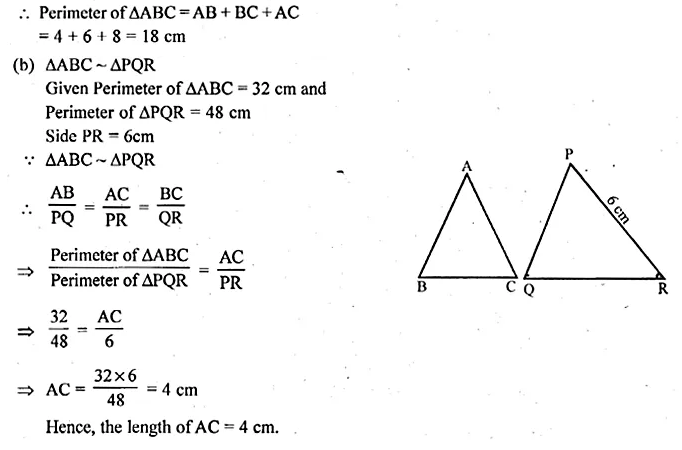Question 7.
Calculate the other sides of a triangle whose shortest side is 6 cm and which is similar to a triangle whose sides are 4 cm, 7 cm and 8 cm.
Solution:
Let ∆ABG ~ ∆DEF in which shortest side of
∆ABC is BC = 6 cm.
In ∆DEF, DE = 8 cm, EF = 4 cm and DF = 7 cm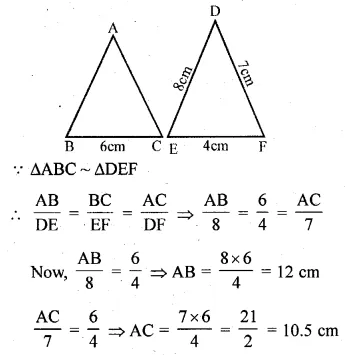Question 8.
(a) In the figure given below, AB || DE, AC = , 3 cm, CE = 7.5 cm and BD = 14 cm. Calculate CB and DC.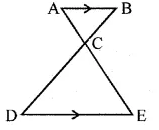(b) In the figure (2) given below, CA || BD, the lines AB and CD meet at G.
(i) Prove that ∆ACO ~ ∆BDO.
(ii) If BD = 2.4 cm, OD = 4 cm, OB = 3.2 cm and AC = 3.6 cm, calculate OA and OC.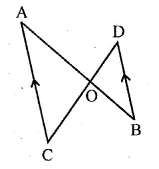Solution:
(a) In the given figure,
AB||DE, AC = 3 cm, CE = 7.5 cm, BD = 14 cm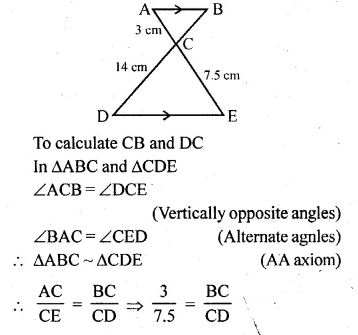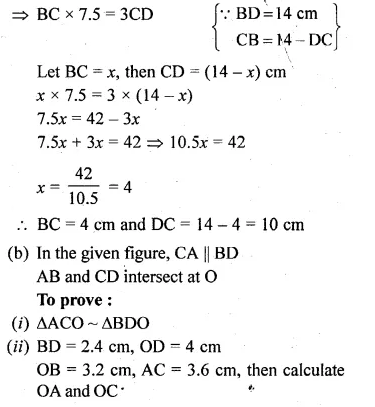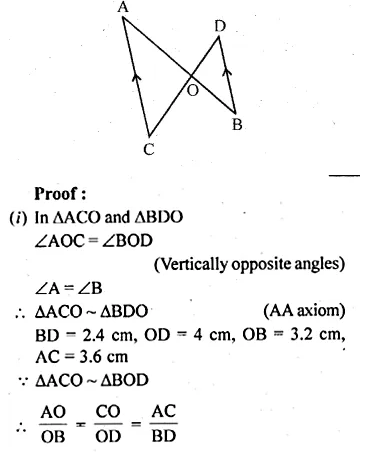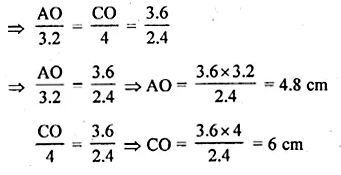Question 9.
(a) In the figure
(i) given below, ∠P = ∠RTS.
Prove that ∆RPQ ~ ∆RTS.
(b) In the figure (ii) given below,
∠ADC = ∠BAC. Prove that CA² = DC x BC.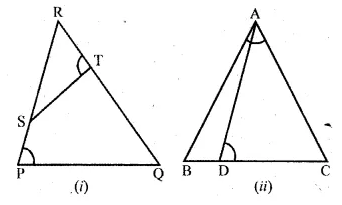Solution:
(a) In the given figure, ∠P = ∠RTS
To prove : ∆RPQ ~ ∆RTS
Proof : In ∆RPQ and ∆RTS
∠R = ∠R (common)
∠P = ∠RTS (given)
∆RPQ ~ ∆RTS (AA axiom)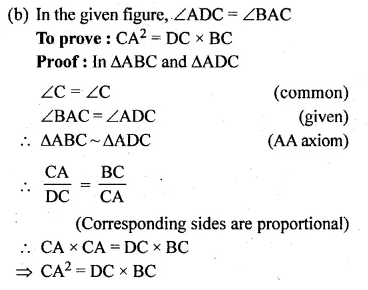Question 10.
(a) In the figure (1) given below, AP = 2PB and CP = 2PD.
(i) Prove that ∆ACP is similar to ∆BDP and AC || BD.
(ii) If AC = 4.5 cm, calculate the length of BD.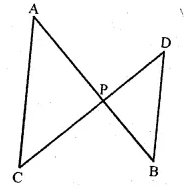(b) In the figure (2) given below,
(i) Prove that ∆s ABC and AED are similar.
(ii) If AE = 3 cm, BD = 1 cm and AB = 6 cm, calculate AC.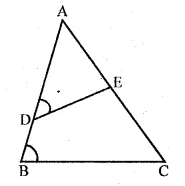(c) In the figure (3) given below, ∠PQR = ∠PRS. Prove that triangles PQR and PRS are similar. If PR = 8 cm, PS = 4 cm, calculate PQ.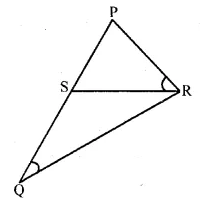Solution:
In the given figure,
AP = 2PB, CP = 2PD
To prove: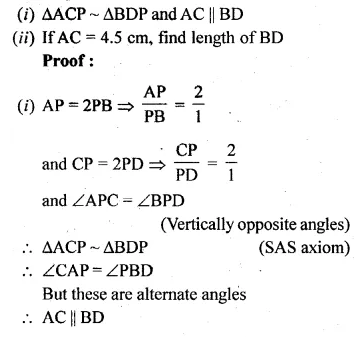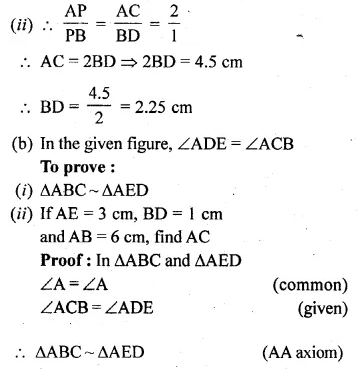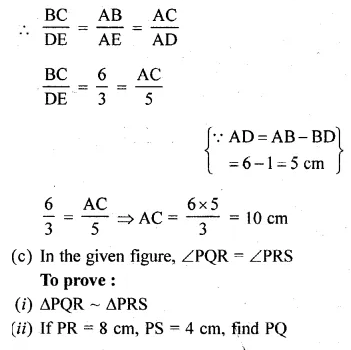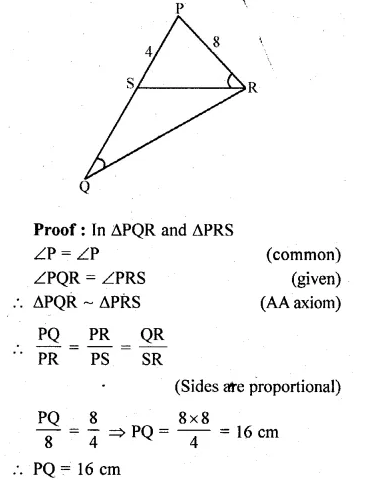Question 11.
In the given figure, ABC is a triangle in which AB = AC. P is a point on the side BC such that PM ⊥ AB and PN ⊥ AC. Prpve that BM x NP = CN x MP.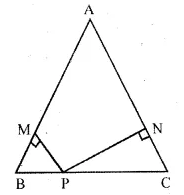Solution:
In the given figure, ABC in which AB = AC.
P is a point on BC such that PM ⊥ AB and PN ⊥ AC
To prove : BM x NP = CN x MPQuestion 12.
Prove that the ratio of the perimeters of two similar triangles is the same as the ratio of their corresponding sides.
Solution:
Given : ∆ABC ~ ∆PQR .
To prove : Ratio in their perimeters k
the same as the ratio in their corresponding sides.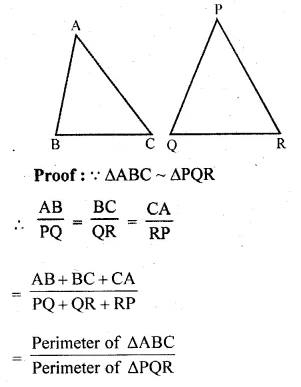Question 13.
In the given figure, ABCD is a trapezium in which AB || DC. The diagonals AC and BD intersect at O. Prove that $$\frac { AO }{ OC } =\frac { BO }{ OD }$$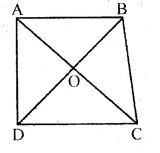Using the above result, find the value(s) of x if OA = 3x – 19, OB = x – 4, OC = x – 3 and OD = 4.
Solution:
ABCD is a trapezium in which AB || DC
Diagonals AC and BD intersect each other at O.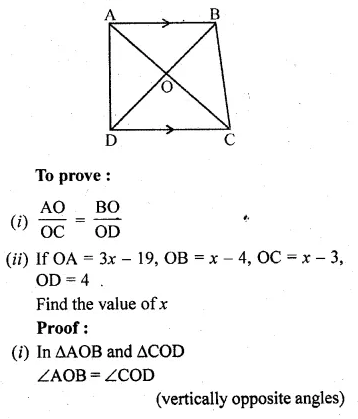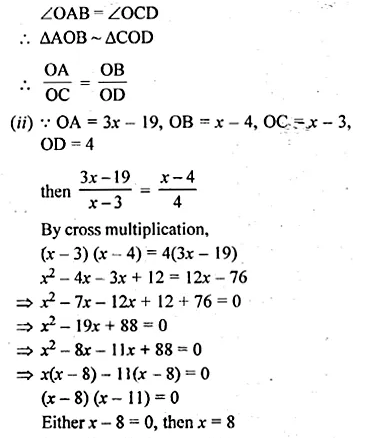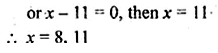Question 14.
In ∆ABC, ∠A is acute. BD and CE are perpendicular on AC and AB respectively. Prove that AB x AE = AC x AD.
Solution:
In ∆ABC, ∠A is acute
BD and CE are perpendiculars on AC and AB respectively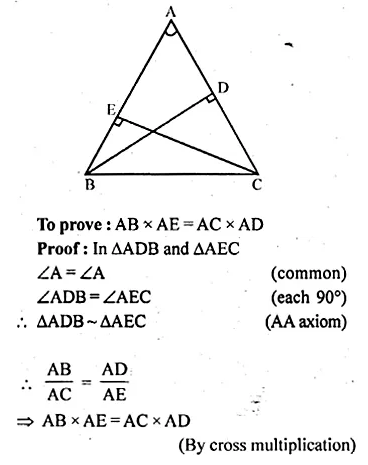Question 15.
In the given figure, DB ⊥ BC, DE ⊥ AB and AC ⊥ BC. Prove that $$\frac { BE }{ DE } =\frac { AC }{ BC }$$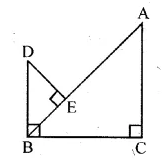Solution:
In the given figure, DB ⊥ BC, DE ⊥ AB and AC ⊥ BC
To prove : $$\frac { BE }{ DE } =\frac { AC }{ BC }$$
Proof: In ∆ABC and ∆DEB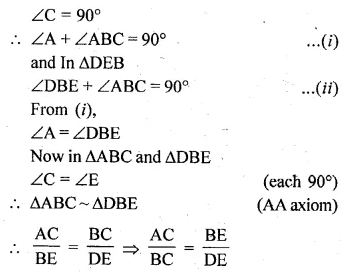Question 16.
(a) In the figure (1) given below, E is a point on the side AD produced of a parallelogram ABCD and BE intersects CD at F. show that ∆ABE ~ ∆CFB.
(b) In the figure (2) given below, PQRS is a parallelogram; PQ = 16 cm, QR = 10 cm. L is a point on PR such that RL : LP = 2 : 3. QL produced meets RS at M and PS produced at N.
(i) Prove that triangle RLQ is similar to triangle PLN. Hence, find PN.
(ii) Name a triangle similar to triangle RLM. Evaluate RM.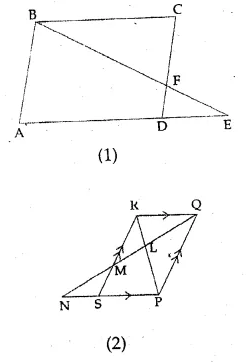Solution:
(a) In the given figure, ABCD is a ||gm
E is a point on AD and produced
and BE intersects CD at F.
To prove : ∆ABE ~ ∆CFB
Proof : In ∆ABE and ∆CFB
∠A = ∠C (opposite angles of a ||gm)
∠ABE = ∠BFC (alternate angles)
∆ABE ~ ∆CFB (AA axiom)
(b) In the given figure, PQRS is a ||gm PQ = 16 cm,
QR = 10 cm
L is a point on PR such that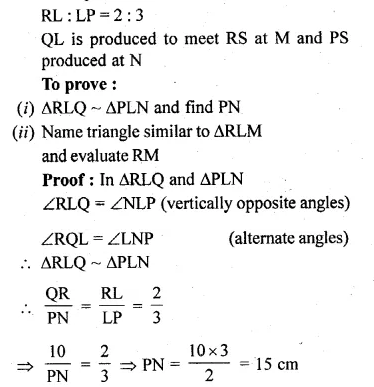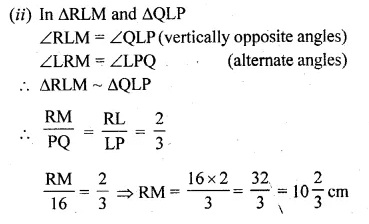Question 17.
The altitude BN and CM of ∆ABC meet at H. Prove that
(i) CN . HM = BM . HN .
(ii) $$\frac { HC }{ HB } =\sqrt { \frac { CN.HN }{ BM.HM } }$$
(iii) ∆MHN ~ ∆BHC.
Solution:
In the given figure, BN ⊥ AC and CM ⊥ AB of ∆ABC
which intersect each other at H.
To prove:
(i) CN.HM = BM.HN
(ii) $$\frac { HC }{ HB } =\sqrt { \frac { CN.HN }{ BM.HM } }$$
(iii) ∆MHN ~ ∆BHC.
Construction: Join MN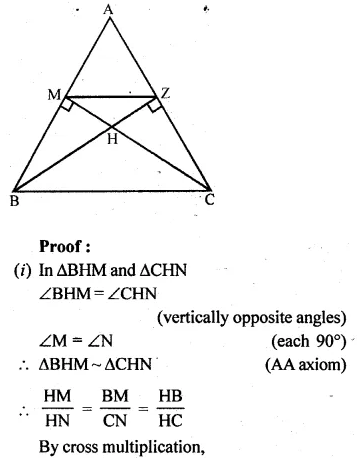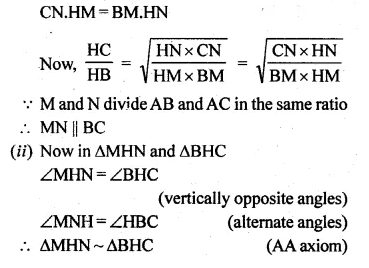Question 18.
In the given figure, CM and RN are respectively the medians of ∆ABC and ∆PQR. If ∆ABC ~ ∆PQR, prove that:
(i) ∆AMC ~ ∆PNR
(ii) $$\frac { CM }{ RN } =\frac { AB }{ PQ }$$
(iii) ∆CMB ~ ∆RNQ.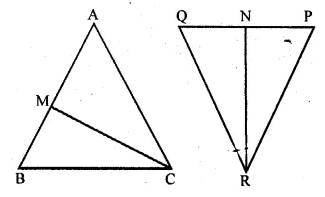Solution:
In the given figure, CM and RN are medians of ∆ABC and ∆PQR
respectively and ∆ABC ~ ∆PQR
To prove:
(i) ∆AMC ~ ∆PNR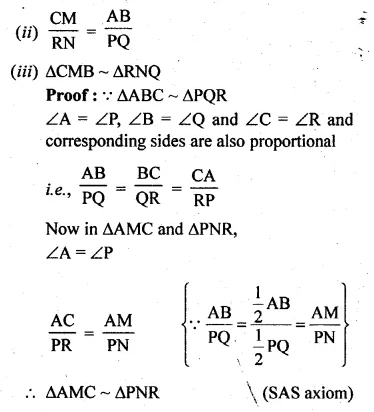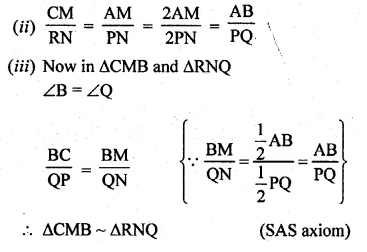>

Question 19.
In the given figure, medians AD and BE of ∆ABC meet at the point G, and DF is drawn parallel to BE. Prove that
(i) EF = FC
(ii) AG : GD = 2 : 1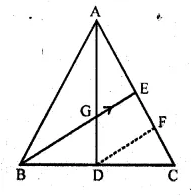Solution:
In the given figure,
AD and BE are the medians of ∆ABC
intersecting each other at G
DF || BE is drawn
To prove :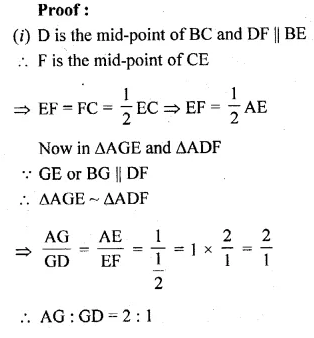Question 20.
(a) In the figure given below, AB, EF and CD are parallel lines. Given that AB =15 cm, EG = 5 cm, GC = 10 cm and DC = 18 cm. Calculate
(i) EF
(ii) AC.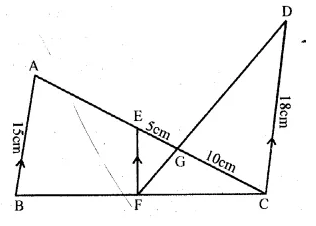(b) In the figure given below, AF, BE and CD are parallel lines. Given that AF = 7.5 cm, CD = 4.5 cm, ED = 3 cm, BE = x and AE = y. Find the values of x and y.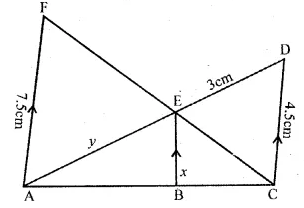Solution:
In the given figure,
AB || EF || CD
AB = 15 cm, EG = 5 cm, GC = 10 cm and DC = 18 cm
Calculate :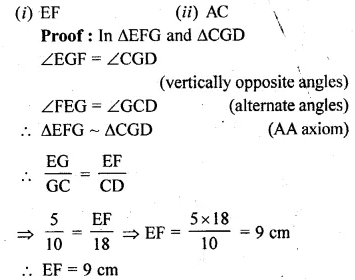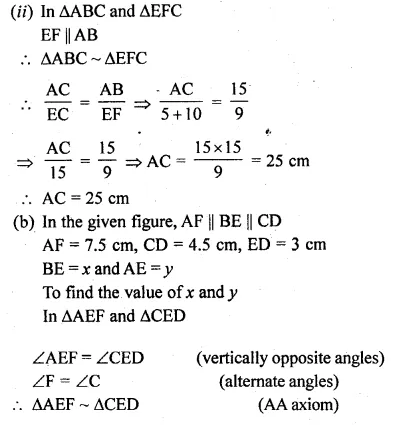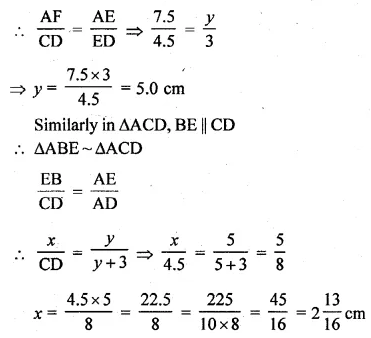Question 21.
In the given figure, ∠A = 90° and AD ⊥ BC If BD = 2 cm and CD = 8 cm, find AD.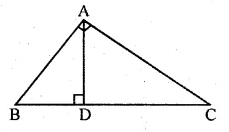Solution:
In ∆ABC, we have ∠A = 90°
Now,
In ∆ABC, we have,
∠BAC = 90°
⇒ ∠BAD + ∠DAC = 90°…..(i)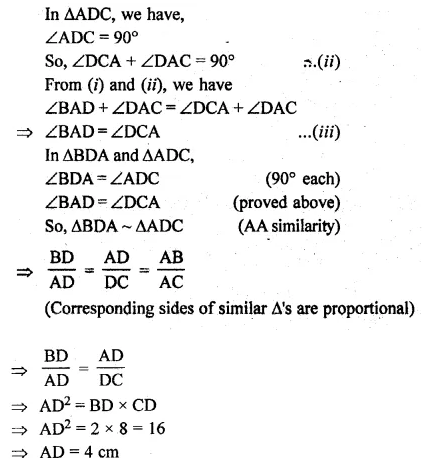Question 22.
A 15 metres high tower casts a shadow of 24 metres long at a certain time and at the same time, a telephone pole casts a shadow 16 metres long. Find the height of the telephone pole.
Solution:
Height of a tower AB = 15 m
and its shadow BC = 24 m
At the same time and position
Let the height of a telephone pole DE = x m
and its shadow EF = 16 m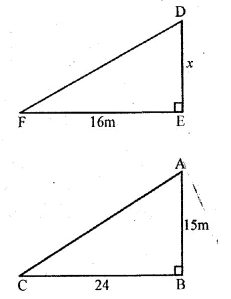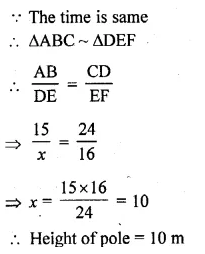Question 23.
A street light bulb is fixed on a pole 6 m above the level of street. If a woman of height casts a shadow of 3 m, find how far she is away from the base of the pole?
Solution:
Height of height pole(AB) = 6m
and height of a woman (DE) = 1.5 m
Here shadow EF = 3 m .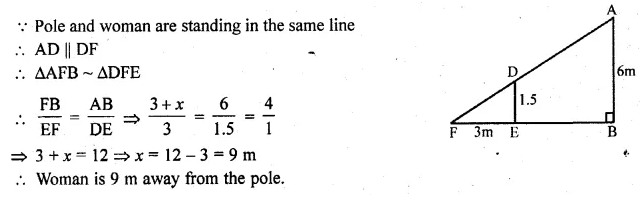Hope given ML Aggarwal Class 10 Solutions for ICSE Maths Chapter 13 Similarity Ex 13.1 are helpful to complete your math homework.

If you have any doubts, please comment below. Learn Insta try to provide online math tutoring for you.